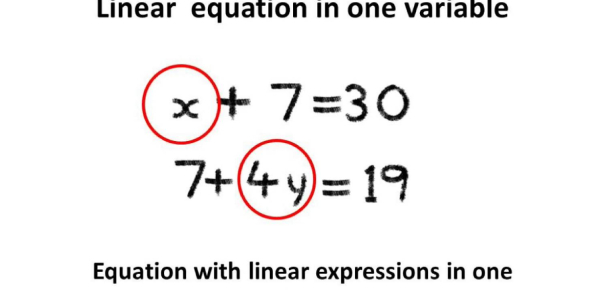# Linear Equations In Two Variables Quiz Questions And Answers

15 Questions | Attempts: 6155SettingsHave you performed well during the linear equations class during school? Let's find it out with linear equations in two variables quiz questions and answers. When it comes to algebra, there are a lot of things to learn. If you think you have learned everything about the two variable linear equations, take this quiz. It will be an easy quiz for you. Just go and ace the quiz! All the best! Do not forget to share the quiz with other mathematicians.

• 1.
The linear equation 4x – 10y = 14 has:
• A.

A unique solution

• B.

Two solutions

• C.

Infinitely many solutions

• D.

No solutions

• 2.
The equation 2x – 3y = 5 has a unique solution if x and y are:
• A.

Natural numbers

• B.

Positive real numbers

• C.

Real numbers

• D.

Rational numbers

• 3.
If (2, 0) is a solution of the linear equation 2x +3y = k, then the value of k is:
• A.

4

• B.

6

• C.

5

• D.

2

• 4.
The graph of the linear equation 2x +3y = 6 cuts the y-axis at the point:
• A.

(2, 0)

• B.

(0, 3)

• C.

(3, 0)

• D.

(0, 2)

• 5.
The equation y = 5, in two variables, can be written as:
• A.

1 . x + 1 . y = 5

• B.

0 . x + 0 . y = 5

• C.

1 . x + 0 . y = 5

• D.

0 . x + 1 . y = 5

• 6.
Any point on the line y = x is of the form:
• A.

(a, –a)

• B.

(0, a)

• C.

(a, 0)

• D.

(a, a)

• 7.
The graph of x = 5 is a line:
• A.

Parallel to the x-axis at a distance 5 units from the origin

• B.

Parallel to the y-axis at a distance 5 units from the origin

• C.

Making an intercept 5 on the x-axis

• D.

Making an intercept 5 on the y-axis

• 8.
x = 9, y = 4 is a solution of the linear equation:
• A.

2x + y = 17

• B.

X + y = 17

• C.

X + 2y = 17

• D.

3x – 2y = 17

• 9.
Any point on the x-axis is of the form:
• A.

(0, y)

• B.

(x, 0)

• C.

(x, x)

• D.

(x, y)

• 10.
If a linear equation has solutions (–3, 3), (0, 0), and (3, –3), then it is of the form:
• A.

Y – x = 0

• B.

X + y = 0

• C.

–2x + y = 0

• D.

–x + 2y = 0

• 11.
The graph of the linear equation 5x + 3y = 10 is a line that meets the x-axis at the point:
• A.

(0, 3)

• B.

(3, 0)

• C.

(2, 0)

• D.

(0, 2)

• 12.
The positive solutions of the equation ax + by + c = 0 always lie in the:
• A.

• B.

• C.

• D.

• 13.
The point of the form (a, –a) always lies on the line:
• A.

X = a

• B.

Y = –a

• C.

Y = x

• D.

X + y = 0

• 14.
The graph of x = 9 is a straight line:
• A.

Intersecting both the axes

• B.

Parallel to y-axis

• C.

Parallel to x-axis

• D.

Passing through the origin

• 15.
The equation of the line parallel to the x-axis and six units above the origin is:
• A.

X = 6

• B.

X = –6

• C.

Y = 6

• D.

Y = –6

## Related TopicsBack to top
×

Wait!
Here's an interesting quiz for you.